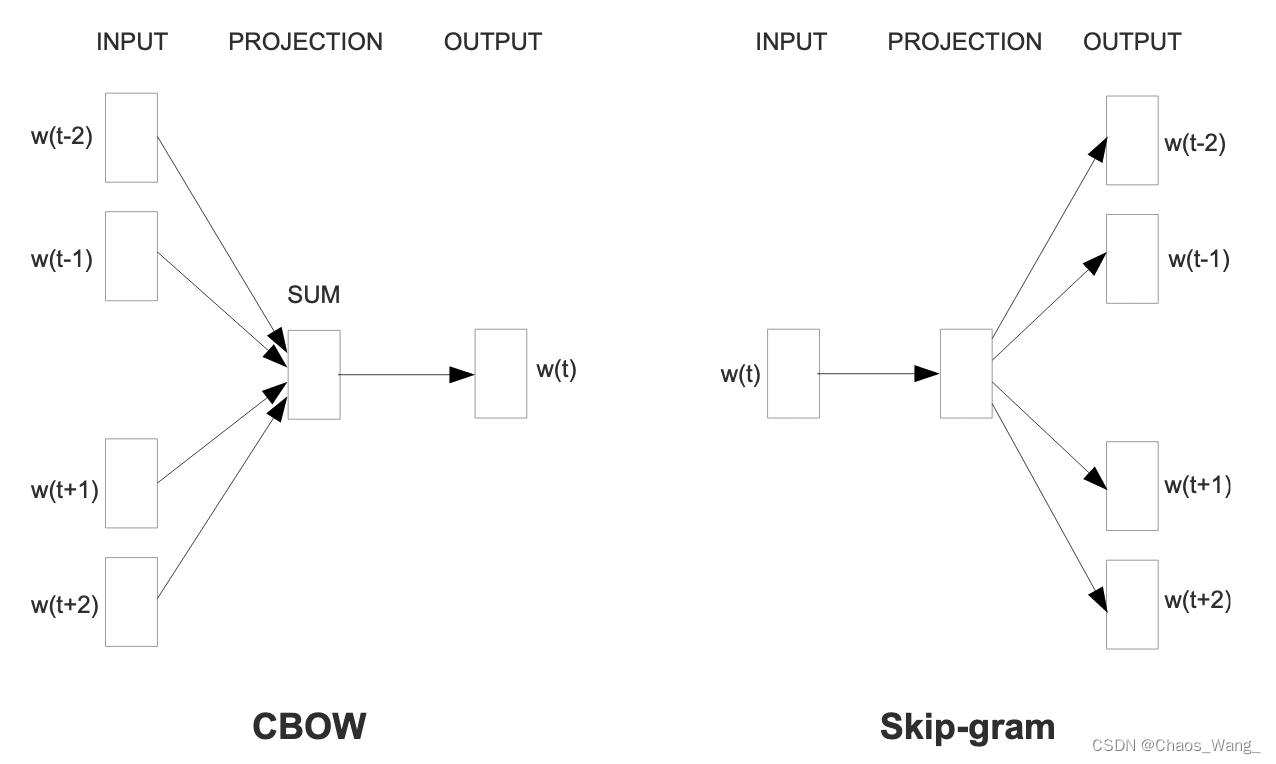## 【NLP经典论文阅读】Efficient Estimation of Word Representations in Vector Space（附代码）

article2023/10/3 19:07:16/

❤️觉得内容不错的话，欢迎点赞收藏加关注😊😊😊，后续会继续输入更多优质内容❤️

👉有问题欢迎大家加关注私戳或者评论（包括但不限于NLP算法相关，linux学习相关，读研读博相关......）👈*（封面图由ERNIE-ViLG AI 作画大模型生成）*

## 1. 论文简介

Efficient Estimation of Word Representations in Vector Space（以下简称Word2vec）是一篇由Google的Tomas Mikolov等人于2013年发表的论文，该论文提出了一种基于神经网络的词向量训练方法，能够高效地学习到单词在向量空间中的分布式表示。

## 2. 论文详解

Word2vec的主要思想是通过预测上下文或目标单词的方法学习单词的向量表示。具体来说，Word2vec通过一个简单的神经网络来学习单词的向量表示，该神经网络包括一个输入层、一个隐藏层和一个输出层。输入层接收到一个单词，将其转换为一个向量表示，然后将该向量传递到隐藏层中。隐藏层对输入向量进行一些变换，然后将结果传递到输出层。输出层则根据上下文或目标单词的不同，采用不同的损失函数来计算损失，然后通过反向传播算法来更新模型参数。

Word2vec有两种模型，分别是CBOW模型和Skip-gram模型。CBOW模型根据上下文单词来预测中心单词，而Skip-gram模型则根据中心单词来预测上下文单词。在训练时，Word2vec通过梯度下降算法来最小化损失函数，并将单词的向量表示作为最终的输出。### 2.1 Skip-gram

L ( θ ) = 1 T ∑ t = 1 T ∑ − c ≤ j ≤ c , j ≠ 0 log ⁡ p ( w t + j ∣ w t ; θ ) L(\theta)=\frac{1}{T}\sum_{t=1}^T\sum_{-c\le j\le c,j\ne 0}\log p(w_{t+j}|w_t;\theta)

p ( w t + j ∣ w t ; θ ) = exp ⁡ ( v w t + j ′ ⋅ v w t ) ∑ i = 1 W exp ⁡ ( v i ′ ⋅ v w t ) p(w_{t+j}|w_t;\theta)=\frac{\exp(v_{w_{t+j}}'\cdot v_{w_t})}{\sum_{i=1}^W\exp(v_i'\cdot v_{w_t})}

### 2.2 CBOW模型

CBOW模型与Skip-gram模型类似，但是反过来。在CBOW模型中，我们的目标是通过上下文单词来预测中心单词。具体来说，我们希望最大化中心单词和其上下下文单词的共现概率，公式如下：
L ( θ ) = 1 T ∑ t = 1 T log ⁡ p ( w t ∣ w t − c , … , w t − 1 , w t + 1 , … , w t + c ; θ ) L(\theta)=\frac{1}{T}\sum_{t=1}^T\log p(w_t|w_{t-c},\ldots,w_{t-1},w_{t+1},\ldots,w_{t+c};\theta)

p ( w t ∣ w t − c , … , w t − 1 , w t + 1 , … , w t + c ; θ ) = exp ⁡ ( ∑ j = − c , j ≠ 0 c v w t + j ) ∑ i = 1 W exp ⁡ ( ∑ j = − c , j ≠ 0 c v w t + j ) p(w_t|w_{t-c},\ldots,w_{t-1},w_{t+1},\ldots,w_{t+c};\theta)=\frac{\exp(\sum_{j=-c,j\ne 0}^cv_{w_{t+j}})}{\sum_{i=1}^W\exp(\sum_{j=-c,j\ne 0}^cv_{w_{t+j}})}

2.3 模型优化

### 2.3 Hierarchical Softmax

p ( w t + j ∣ w t ) = exp ⁡ ( v w t + j T ⋅ v w t ) ∑ i = 1 W exp ⁡ ( v i T ⋅ v w t ) = exp ⁡ ( score ( w t + j , w t ) ) ∑ i = 1 W exp ⁡ ( score ( w i , w t ) ) p(w_{t+j}|w_t)=\frac{\exp(v_{w_{t+j}}^T\cdot v_{w_t})}{\sum_{i=1}^W\exp(v_i^T\cdot v_{w_t})}=\frac{\exp(\text{score}(w_{t+j},w_t))}{\sum_{i=1}^W\exp(\text{score}(w_i,w_t))}

### 2.4 Negative Sampling

P sample ( w ) = f ( w ) 3 / 4 ∑ i = 1 W f ( w i ) 3 / 4 P_{\text{sample}}(w)=\frac{f(w)^{3/4}}{\sum_{i=1}^{W}f(w_i)^{3/4}}

− log ⁡ σ ( v w O ′ ⋅ v w I ) − ∑ k = 1 K log ⁡ σ ( − v w k ′ ⋅ v w I ) -\log\sigma(v'_{w_O}\cdot v_{w_I})-\sum_{k=1}^{K}\log\sigma(-v'_{w_k}\cdot v_{w_I})

## 3. 代码实现

import gensim
from gensim.models import Word2Vec

# 加载语料库
sentences = gensim.models.word2vec.Text8Corpus('path/to/corpus')

# 训练模型
model = Word2Vec(sentences, size=100, window=5, min_count=5, workers=4)

# 保存模型
model.save('path/to/model')

# 加载模型

# 获取单词向量
vector = model['word']


#### 相关文章

coco数据集annotation的segmentation并不是二值mask&#xff0c;而是polygon格式&#xff0c; 看一个annotation. {"segmentation": [[510.66,423.01,511.72,420.03,510.45......]], #两两组成(x,y)坐标&#xff0c;polygon格式"area": 702.1057499999998…

### mac 把word公式默认字体Cambria Math换成LaTex字体以及带章节自动编号

word默认是Cambria Math&#xff0c;想用latex那种公式的字体&#xff0c;这里使用的是XITS Math字体 搜了很多地方&#xff0c;都是用ab Text这个方法先转成文本&#xff0c;再换字体&#xff0c;然后设置斜体 可是公式多起来的话这种办法很麻烦&#xff0c;而且一个公式里常…

### Java实习生------Redis哨兵机制详解⭐⭐⭐

“无数的我们被世界碾压成一缩黑团&#xff0c;无数的我们试图与世界抗争到底”&#x1f339; 参考资料&#xff1a;图解redis 目录 什么是哨兵机制&#xff1f; 哨兵机制主要干了哪三件事&#xff1f; 哨兵监控主节点的过程是怎样的&#xff1f; 判断主节点故障之后&…

### 垃圾回收之CMS、G1、ZGC对比

ZGC&#xff08;The Z Garbage Collector&#xff09;是JDK 11中推出的一款低延迟垃圾回收器&#xff0c;它的设计目标包括&#xff1a; 停顿时间不超过10ms&#xff1b;停顿时间不会随着堆的大小&#xff0c;或者活跃对象的大小而增加&#xff1b;支持8MB~4TB级别的堆&#x…